Your browser does not support the IFRAME tag.Home MonkeyNotes Printable Notes Digital Library Study Guides Message Boards Study Smart Parents Tips College Planning Test Prep Fun Zone Help / FAQ How to Cite New Title Request

 8.3 The cuboid and the cube A book, a match box, a brick are all examples of a cuboid. The definition of a cuboid is derived from that of the prism. A cuboid is a solid formed by joining the corresponding vertices of two congruent rectangles such that the lateral edges are perpendicular to the planes containing the congruent rectangles. Figure 8.3 shows a cuboid.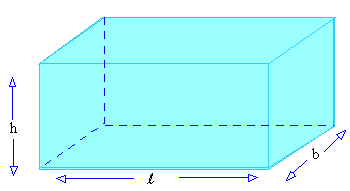Figure 8.3 As can be seen in Figure 8.3 the cuboid has six surfaces and each one is congruent and parallel to the one opposite to it. Thus there is a pair of three rectangles which goes to make a cube. It is already known that the area of a rectangle is the product of its length and breadth, l ´ b = Area. The surface area of a cuboid is the sum of the areas of all its faces,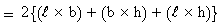Now consider a cuboid made of 6 congruent squares instead of a pair of three rectangles. This structure has l = b = h i.e. the length breadth and height are the same. Such a cuboid is called a cube. Cube A cube is a square right prism with the lateral edges of the same length as that of a side of the base. (See figure 8.4)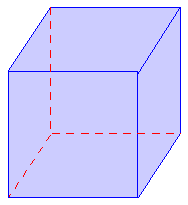Figure 8.4 As can be seen in Figure 8.4 in a cube the length, breadth and height are equal. Therefore the surface of a cube is essentially six squares of equal length. The area of a square is the square of its length. The total surface area of the cube is the sum of the areas of all its 6 surfaces. If length of one side of the cube is l the area of one surface is l 2 \ the surface area of the cube = 6 ´ l 2 = 6 l 2 . To find volume of a cuboid first a unit cube has to be defined. A unit cube is a cube with length equal to one unit. This unit is chosen as per convenience. For small volumes 1 cm can be chosen as the unit length . The volume of this cube is 1 cubic cm. It is represented as 1 cm3. For larger volumes 1 meter can be used as the unit length. In this case the volume of the unit cube is 1 cubic meter and is represented by 1m3 . Depending on the size of the cuboid whose volume is to be measured the appropriate unit cube is chosen. The volume of a cuboid is the number of unit cubes that are required to fill the cuboid completely. Figure 8.6 shows a cuboid whose volume is to be measured. This cube has length 10 cm breadth 8 cm and height 4 cm. The three edges OA, OB and OC are perpendicular to each other. On these sides points LM and N are chosen such that l (seg. O l) = l (seg. ON) = unit length.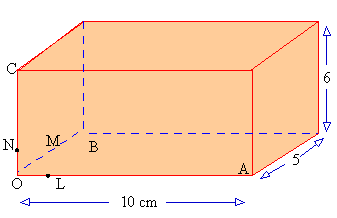Figure 8.5 These sides seg. OL, seg. OM and seg. ON form three sides of a unit cube as shown in Figure 8.6a Let another unit cube be placed on the right side of this unit cube such that one of its edges lies along seg. LA and one of its face covers the right side of the first unit cube. (See Figure 8.6b)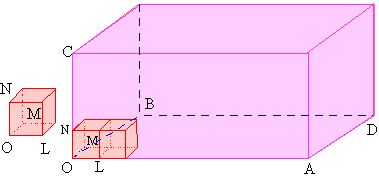Figure 8.6a                       Figure 8.6b Since length of seg. OA = 10 and l (seg. OL) = 1, 10 unit cubes can be arranged along OA. Similarly l (seg. OB) = 5. \ 5 unit cubes can be arranged along seg. OB. Thus to cover the face AOBD, 10 ´ 5 = 50 unit cubes will be required. They will form the first layer towards filling the cuboid. Since l (seg. OC) = 6 it is obvious that 6 such layers are required to fill the cuboid. \ Number of unit cubes required = 50 ´ 6  = 300 Thus the space occupied by the cuboid or its volume = 300 cubic units = 300 unit3 In general it may be stated that for a cuboid with length l , breadth b and height h the volume = l ´ b ´ h cubic units. For a cuboid, Surface area = 2 (l b + b h + l h) Volume = (l ´ b ´ h) cubic units. Since a cube has l = b = c its surface area = 6 l 2 & Volume = l 3 Index 8.1 Introduction to solid geometry 8.2 Prism 8.3 The cuboid and the cube 8.4 Cylinders 8.5 Pyramids 8.6 Right circular cone 8.7 Sphere Chapter 9
 Search: All Products Books Popular Music Classical Music Video DVD Toys & Games Electronics Software Tools & Hardware Outdoor Living Kitchen & Housewares Camera & Photo Cell Phones Keywords: